# Solving Linear Equations

• Introduction

• We remember that...

• 15 resolved linear equations step by step

## Introduction

In mathematics, linear equations are the introduction to algebra. Their understanding is vital for any kind of equations: quadratic equations or bigger, exponentials, irrational, etc. and for equation systems.

In real life, although it may not be though of straight away, but equations are a very useful tool that allows us to resolve problems that we face on a day to day basis. We can see this by going to the linear equations problems section.

These equations are known as linear, because the monomial literal part does not have an exponent (for example, $$3x$$ can be part of a linear equation, but $$3x^2$$ cannot because it is quadratic), so represented in a chart appears as a straight line. This fact assures us that if there is a solution, there can only be one solution (except in special cases where there are infinite solutions).

We say if there is a solution because sometimes equations don not any solution. For example, the equation $$x = x + 1$$ (which means a number equals the consecutive number) does not have a solution, because this is never true. Actually, this equation is reduced to 1 = 0, which is impossible.

In this section we resolve linear equations with an increasing difficulty: simple equations, equations with fractions (where we will use the least common multiple of the denominators), equations with parenthesis and equations with nested parenthesis (parenthesis within parenthesis).

Similar pages:

## We remember that...

• If we obtain an impossible equality, there is no solution, like 1 = 0.

• If we obtain an equality that is always true, whatever value gives a solution. Hence the solution is all the real numbers. For example, if we obtain 0 = 0.

• When there are denominators and we want to avoid them, we multiply the full equation by the same least common multiple of the denominators. Hence the denominators disappear.

• To remove the parenthesis, we multiply the coefficient previous to the parenthesis by all the elements it contains. This coefficient can be a negative sign (like –1, the content changes sign), a positive sign (like +1, the content does not change) or a positive or negative number or fractions (this number multiplies everything in the parentheses, changing the signs whenever it is negative).

• When we have a nested parenthesis, a parenthesis inside another parenthesis, we begging removing from outside to the inside. First, we remove the exterior parenthesis (multiplying their content by the coefficients) and afterwards, we remove the rest the same way: from the most exterior to the most interior.

Realistically, there is not any need to follow an order when removing the parentheses, but it is recommended to follow one when we are learning.

# 15 Resolved Linear Equations

Equation 1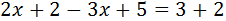Show solution

Equation 2Show solution

Equation 3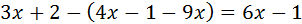Show solution

Equation 4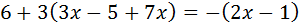Show solution

Equation 5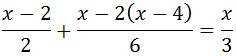Show solution

Equation 6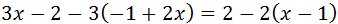Show solution

Equation 7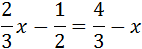Show solution

Equation 8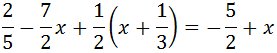Show solution

Equation 9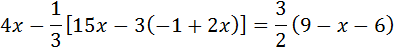Show solution

Equation 10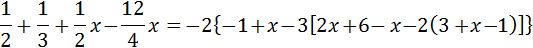Show solution

Equation 11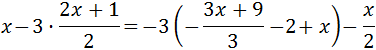Show solution

Equation 12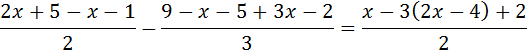Show solution

Equation 13Show solution

Equation 14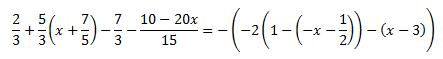Show solution

Equation 15Show solution